# Solution assignment 03 Tangent line to graph

### Assignment 3

Calculate the slope of the tangent line at the point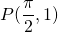of the graph of the function: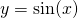### Solution

First we calculate the derivative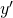: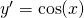In the point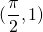the derivative has the value: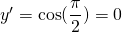and thus the slope of the tangent line at:equals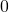.